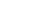Why You Should Use A Home Loan Calculator Before Applying For A LoanBuying home is a dream that we all have and with the help of home loan it has become bit easy to fulfil this dream. There are an important terminologies associated with home loan i.e. “EMI” (Equated Monthly Instalments). It can either be paid through auto-debit instructions set up on your salary account or you can send cheques every month. In either scenario, the amount to be paid back is the same, month on month, for the entire tenure.

Formula for home loan calculator

EMI = [P x R x (1+R)^N]/[(1+R)^N-1],

In this formula, the letters stand for:

EMI is the equated monthly instalment

P is the principal or the amount that is borrowed as a loan

R is the rate of interest that is levied on the loan amount (the interest rate should be a monthly rate)

N is the tenure of repayment of the loan or the number of monthly instalments that you will pay (tenure should be in months)

It is not easy to calculate EMI applicable on your home loan using Excel or by hand, as it is a complicated one. Hence, it is best to use a home loan EMI calculator for the same. Using a housing loan calculator you can calculate the EMI within seconds and save yourself from time-consuming tedious calculations.

How it works Home loan EMI calculator computes the EMI according to the details entered by you in the calculator. The EMI calculator for home loans works on the following inputs:

Loan Amount: This is the sum amount that you want to borrow for purchasing your house. This amount is mostly depend upon the cost of the property as well as your repayment capacity. Bank or financial institution can only provide 80% of the property value as loan. This is the principal amount of the loan and the rate of interest is applied to it. The final amount (post-levy of the interest rate) is then divided equally by the tenure of the loan. You can enter the amount you wish to borrow into the EMI calculator. You can enter the EMI or monthly instalment keeping in mind that it should not be more than 40% of your monthly income.

Interest Rate: It is the rate at which interest is levied on the amount lent. It differs from one financial institution to another, so it is best to check the interest rates before applying for a home loan. You can opt for a fixed rate of interest or floating rate of interest. In fixed Interest rate, the interest rate remains unchanged for the entire loan tenure and all loan EMIs are equal. In the case of floating interest rate, the rates will fluctuate with any change in the base rate and hence the EMIs vary.

Tenure: It is the time allotted to or chosen for repayment of the loan. Most of the loans come with flexible loan tenures. Home loans are have a longer repayment tenure at times even up to 30 years. The longer the tenure you choose the more interest you will have to pay.